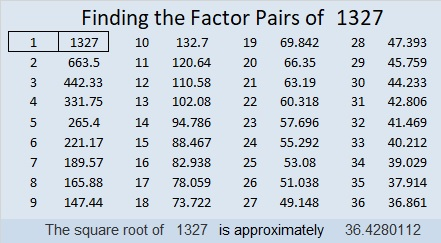# 1327 Record Breaking Prime Gap

Here is a table of prime numbers with the gaps between them and the next prime number. When a prime gap is larger than any previous prime gap, I’ve highlighted it in yellow. As you can see 1327 breaks the previous record of 22 held by 1129.That the next 33 numbers after 1327 are all composite is pretty remarkable. Even more remarkable is the fact that 1327 will hold the record for being the smallest prime number with the biggest prime gap until 9551 breaks it with a slightly larger prime gap of 36.

Here is some additional information about the number 1327:

• 1327 is a prime number.
• Prime factorization: 1327 is prime.
• The exponent of prime number 1327 is 1. Adding 1 to that exponent we get (1 + 1) = 2. Therefore 1327 has exactly 2 factors.
• Factors of 1327: 1, 1327
• Factor pairs: 1327 = 1 × 1327
• 1327 has no square factors that allow its square root to be simplified. √1327 ≈ 36.42801

How do we know that 1327 is a prime number? If 1327 were not a prime number, then it would be divisible by at least one prime number less than or equal to √1327 ≈ 36.4. Since 1327 cannot be divided evenly by 2, 3, 5, 7, 11, 13, 17, 19, 23, 29 or 31, we know that 1327 is a prime number.